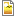Total float vs free float Oliver Lehman question 18

Hi,
Just took Oliver Lehmans 75 free preparation questions. I have a problem understanding the answer to q. 18 Use link for the total question including the necessary network diagram or look in my attachment.
http://www.oliverlehmann.com/pmp-self-test/75-free-questions.htm18. Start dates in the following network logic diagram are defined as early morning, finish dates are evening.
If tasks are scheduled to begin at early start date, what is true?

Activity B has a free float of 10 d.
Activity B has a total float of 10 d.
Activity A has a free float of 10 d.
Activity A has a total float of 10 d.

This is how I calculated:I get total float for activity B to be equal to 10 days (LS=26, ES=16, Total float 26-16=10 days) and free float for activity be also to be equal to 10 days (ES of next activity=31, EF of activity B=21, Free float 31-21= 10 days.

Correct answer is B (total float=10 days) But how do I know that it is not A?

AttachmentSizeQ 18 total vs free float.png92.38 KB

You're right, both A & B

You're right, both A & B options are correct.

As per my understanding, "Total Float" in schedule network diagram is calculated first and then "Free Float" so considering both options are correct and limited information is available in question, Answer related to Total Float should get higher priority.

Thanks!

OK! Thanks! I can believe that to be a reasonable explanation.

My 2 cents

Hello

I was just about to post the same question, before I saw this. Doesnt it make you mad to see one question having two possible answers and the confusion surrounding it, (as if the whole pmp prep thing isnt taxing enough? :)). I researched about the same topic on how to calculate the free float online, funny another posting on the same topic brought about a discussion, where there were so many interpretations of how to calculate the free float. Anyways, I found this link

http://pmp-study.blogspot.com/2009/08/tips-for-critical-path-total-float.html

I believe that the free float is calculated as mentioned: ES(successor activity D) - EF (current activity B) -1 = 31-21-1 = 9. I used '-1' to calculate this, because the first activity A starts on day1. If for any reason it started with a value 0 it would be  ES(successor activity D) - EF (current activity B) = 10.

question 18

The -1 explanation doesnt really fit because any delay in activity D is a delay to the critical path.

this just seems like a bad sample exam question because A and B are correct and there is no "more correct" answer in this situation.